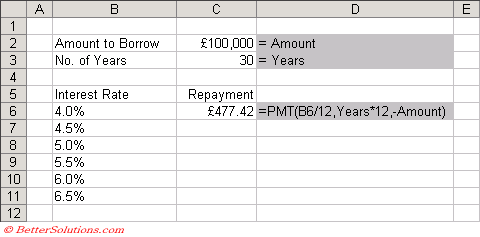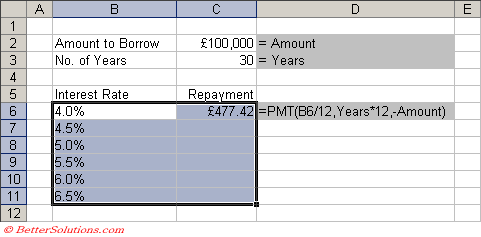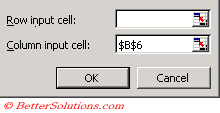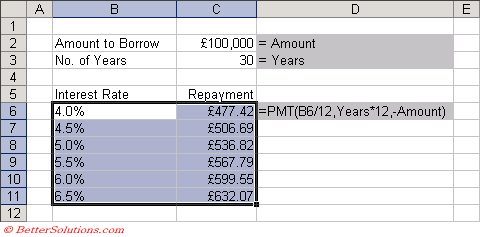# One Variable

A data table summarises the impact of one variable on a formula.
Lets consider a simple mortgage calculation.
Suppose you are looking to buy a house and you want to borrow £100,000 to be repayed over 30 years.
You may want to work out what the different monthly repayments will be given different interest rates.
This type of problem can be solved by using a one variable data table.

### Create a Simple Table

You must create a table that contains at least two columns and two rows.
The first column should contain the values for one of the arguments you want to substitute in the formula.
The second column must contain the formula in the first cell.
Create a two column table that contains various interest rates in one column and the monthly repayment in the other.
The worksheet function that can be used to calculate the payment for a loan given a fixed interest rate is the PMT function.
Create the following table and the following named ranges:
"C2" = Amount
"C3" = YearsEnter the formula to calculate the payment amount into cell "C6".
This formula will calculate the monthly repayment using a 4.0% interest rate.
The monthly repayment with an interest rate of 4.0% is £477.42.

### Using Data Table

Highlight both the columns, so in this case the range of cells "B6:C11".
You do not need to include any column or row headings.
The first column contains the values for the argument you want to substitute in the formula.
The second column contains the formula which refers to this first column.Select (Data > Table) to display the Table dialog box.
Enter the first cell from the column you want to substitue into the "Column input cell". In this case cell "B6".Press OK and the remaining cells in the second column will be automatically populated using the formula provided.The formula is not copied to all the cells but a special array formula is used instead "{=TABLE(,B6)}".

What is the formula ?? {=TABLE() }
To create a one-variable data table, enter the input values into a column or row include an empty cell as a reference cell ?
and enter the formula for which you want to calculate the results
Then use the data table tool to identify the reference cell and Excel automatically uses the TABLE function to calculate the result set

### One Input Variable in a Row

If you are using rows instead of columns then the substitution values must be in the first row.
When you create a formula the argument you want to substitute must be in the first column of the table.
Remember to enter the first cell from the row you want to substitute into the "Row input cell".

### Important

When you are using a one variable data table you do not need to enter a cell into both the "Column input cell" and the "Row input cell".
When you highlight the table do not include the column or row headings.
The data table has one advantage over a pivot table and that is that it is updated automatically and does not need to be refreshed.